## Sunday, February 1, 2009

### AC Generation Analysis

Analysis of the AC power generation process and of the alternating current we use in almost every aspect of our lives is necessary to better understand how AC power is used in today’s technology.

Effective Values

The output voltage of an AC generator can be expressed in two ways. One is graphically by use of a sine wave (Figure 1). The second way is algebraically by the equation e = Emax sin wt, which will be covered later in the text.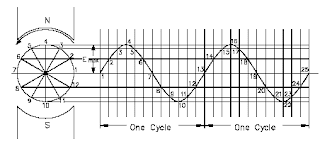Figure 1 Voltage Sine Wave

When a voltage is produced by an AC generator, the resulting current varies in step with the voltage. As the generator coil rotates 360°, the output voltage goes through one complete cycle. In one cycle, the voltage increases from zero to Emax in one direction, decreases to zero, increases to Emax in the opposite direction (negative Emax), and then decreases to zero again. The value of Emax occurs at 90° and is referred to as peak voltage. The time it takes for the generator to complete one cycle is called the period, and the number of cycles per second is called the frequency (measured in hertz).

One way to refer to AC voltage or current is by peak voltage (Ep) or peak current (Ip). This is the maximum voltage or current for an AC sine wave.

Another value, the peak-to-peak value (Ep-p or Ip-p), is the magnitude of voltage, or current range, spanned by the sine wave. However, the value most commonly used for AC is effective value. Effective value of AC is the amount of AC that produces the same heating effect as an equal amount of DC. In simpler terms, one ampere effective value of AC will produce the same amount of heat in a conductor, in a given time, as one ampere of DC. The heating effect of a given AC current is proportional to the square of the current. Effective value of AC can be calculated by squaring all the amplitudes of the sine wave over one period, taking the average of these values, and then taking the square root. The effective value, being the root of the mean (average) square of the currents, is known as the root-mean-square, or RMS value. In order to understand the meaning of effective current applied to a sine wave, refer to Figure 2.

The values of I are plotted on the upper curve, and the corresponding values of I^2 are plotted on the lower curve. The I2 curve has twice the frequency of I and varies above and below a new axis. The new axis is the average of the I^2 values, and the square root of that value is the RMS, or effective value, of current. The average value is ½ Imax^2. The RMS value is then, 2^(1/2)Imax^2/ 2 or 2^(1/2)/2 x Imax, which is equal to 0.707 Imax.
There are six basic equations that are used to convert a value of AC voltage or current to another value, as listed below.

1. Average value = peak value x 0.637……………….(1)
2. Effective value (RMS) = peak value x 0.707………(2)
3. Peak value = average value x 1.57…………………(3)
4. Effective value (RMS) = average value x 1.11…….(4)
5. Peak value = effective value (RMS) x 1.414………(5)
6. Average value = effective (RMS) x 0.9……………(6)

The values of current (I) and voltage (E) that are normally encountered are assumed to be RMS values; therefore, no subscript is used.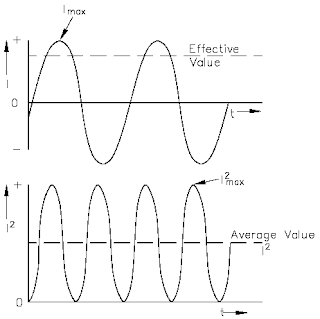Figure 2 Effective Value of Current

Another useful value is the average value of the amplitude during the positive half of the cycle.
Equation (7) is the mathematical relationship between Iav , Imax , and I.

Iav = 0.637 Imax = 0.90 I………….(7)

Equation (8) is the mathematical relationship between Eav , Emax , and E.

Eav = 0.637 Emax = 0.90 E……….(8)

Example 1: The peak value of voltage in an AC circuit is 200 V. What is the RMS value of the voltage?
Solution:
E = 0.707 Emax = 0.707 (200 V) = 141.4 V

Example 2: The peak current in an AC circuit is 10 amps. What is the average value of current in the circuit?
Solution:
Iav = 0.637 Imax = 0.637 (10 amps) = 6.37 amps

Phase Angle

Phase angle is the fraction of a cycle, in degrees, that has gone by since a voltage or current has passed through a given value. The given value is normally zero. Referring back to Figure 1, take point 1 as the starting point or zero phase. The phase at Point 2 is 30°, Point 3 is 60°, Point 4 is 90°, and so on, until Point 13 where the phase is 360°, or zero. A term more commonly used is phase difference. The phase difference can be used to describe two different voltages that have the same frequency, which pass through zero values in the same direction at different times. In Figure 3, the angles along the axis indicate the phases of voltages e1 and e2 at any point in time. At 120°, e1 passes through the zero value, which is 60° ahead of e2 (e2 equals zero at 180°). The voltage e1 is said to lead e2 by 60 electrical degrees, or it can be said that e2 lags e1 by 60 electrical degrees.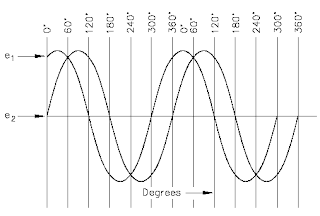Figure 3 Phase Relationship

Phase difference is also used to compare two different currents or a current and a voltage. If the phase difference between two currents, two voltages, or a voltage and a current is zero degrees, they are said to be "in-phase." If the phase difference is an amount other than zero, they are said to be "out-of-phase."

Voltage Calculations

Equation (9) is a mathematical representation of the voltage associated with any particular orientation of a coil (inductor).

e = Emax sin teta........(9)

where
e = induced EMF
Emax = maximum induced EMF
 (teta)= angle from reference (degrees or radians)

Example 1: What is the induced EMF in a coil producing a maximum EMF of 120 V when the angle from reference is 45°?
e = Emax sin teta= 120 V (sin 45°)= 84.84 V

The maximum induced voltage can also be called peak voltage Ep. If (t) is the time in which the coil turns through the angle, teta (), then the angular velocity, w () of the coil is equal to teta/t and is expressed in units of radians/sec. Equation (10) is the mathematical representation of the angular velocity.
teta = wt………….(10)

where
t = time to turn through the angle from reference (sec)

Using substitution laws, a relationship between the voltage induced, the maximum induced voltage, and the angular velocity can be expressed. Equation (11) is the mathematical representation of the relationship between the voltage induced, the maximum voltage, and the angular velocity, and is equal to the output of an AC Generator.

e = Emax sin (wt)……………(11)

where
e = induced EMF (volts)
Emax = maximum induced EMF (volts)
, w = angular velocity (radians/sec)
t = time to turn through the angle from reference (sec)

Current Calculations

Maximum induced current is calculated in a similar fashion. Equation (7-12) is a mathematical representation of the relationship between the maximum induced current and the angular velocity.

i = Imax sin (wt)…………(12)

where
i = induced current (amps)
Imax = maximum induced current (amps)
t = time to turn through the angle from reference (sec)

Frequency Calculations

The frequency of an alternating voltage or current can be related directly to the angular velocity of a rotating coil. The units of angular velocity are radians per second, and 2radians is a full revolution. A radian is an angle that subtends an arc equal to the radius of a circle. One radian equals 57.3 degrees. One cycle of the sine wave is generated when the coil rotates 2phi (2 x 3.14) radians. Equation (13) is the mathematical relationship between frequency (f) and the angular velocity, w () in an AC circuit.

w = 2phi.f --> 2 x 3.14 x f………….(13)
where
f = frequency (HZ)

Example 1: The frequency of a 120 V AC circuit is 60 Hz. Find the following:
1. Angular velocity
2. Angle from reference at 1 msec
3. Induced EMF at that point

Solution:
1. w= 2phi.f = 2 (3.14) (60 Hz) = 376.8 radians/sec
2. teta = wt = (376.8 radian/sec) (.001 sec) = 0.3768 radians
3. e = Emax sin teta= (120 V) (sin 0.3768 radians)= (120 V) (0.3679)= 44.15 V

Summary

Voltage, Current, and Frequency Summary

• The following terms relate to the AC cycle: radians/second, the velocity the loop turns; hertz, the number of cycles in one second; period, the time to complete one cycle.
• Effective value of AC equals effective value of DC.
• Root mean square (RMS) values equate AC to DC equivalents:
- I = 0.707 Imax = Effective Current
- E = 0.707 Emax = Effective Voltage
- Iav = 0.636 Imax = 0.9 I = Average Current
- Eav = 0.636 Emax = 0.9 E = Average Voltage
• Phase angle is used to compare two wave forms. It references the start, or zero point, of each wave. It compares differences by degrees of rotation. Wave forms with the same start point are "in-phase" while wave forms "out-of-phase" either lead or lag.

### AC Generation

An understanding of how an AC generator develops an AC output will help us analyze the AC power generation process.

The elementary AC generator (Figure 1) consists of a conductor, or loop of wire in a magnetic field that is produced by an electromagnet. The two ends of the loop are connected to slip rings, and they are in contact with two brushes. When the loop rotates it cuts magnetic lines of force, first in one direction and then the other.

Development of a Sine-Wave Output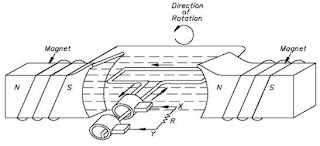Figure 1 Simple AC Generator

At the instant the loop is in the vertical position (Figure 2, 0o), the coil sides are moving parallel to the field and do not cut magnetic lines of force. In this instant, there is no voltage induced in the loop. As the coil rotates in a counter-clockwise direction, the coil sides will cut the magnetic lines of force in opposite directions. The direction of the induced voltages depends on the direction of movement of the coil. The induced voltages add in series, making slip ring X (Figure 1) positive (+) and slip ring Y (Figure 1) negative (-). The potential across resistor R will cause a current to flow from Y to X through the resistor. This current will increase until it reaches a maximum value when the coil is horizontal to the magnetic lines of force (Figure 2, 90o). The horizontal coil is moving perpendicular to the field and is cutting the greatest number of magnetic lines of force. As the coil continues to turn, the voltage and current induced decrease until they reach zero, where the coil is again in the vertical position (Figure 2, 180o). In the other half revolution, an equal voltage is produced except that the polarity is reversed (Figure 2, 270o, 360o). The current flow through R is now from X to Y (Figure 1).Figure 2 Developing a Sine-Wave Voltage

The periodic reversal of polarity results in the generation of a voltage, as shown in Figure 2. The rotation of the coil through 360° results in an AC sine wave output.

Summary

• A simple generator consists of a conductor loop turning in a magnetic field, cutting across the magnetic lines of force.
• The sine wave output is the result of one side of the generator loop cutting linesof force. In the first half turn of rotation this produces a positive current and in the second half of rotation produces a negative current. This completes one cycle of AC generation.

### DC Circuit Terminology

Before operations with DC circuits can be studied, an understanding of the types of circuits and common circuit terminology associated with circuits is essential.

Schematic Diagram

Schematic diagrams are the standard means by which we communicate information in electrical and electronics circuits. On schematic diagrams, the component parts are represented by graphic symbols, some of which were presented earlier in Module 1. Because graphic symbols are small, it is possible to have diagrams in a compact form. The symbols and associated lines show how circuit components are connected and the relationship of those components with one another.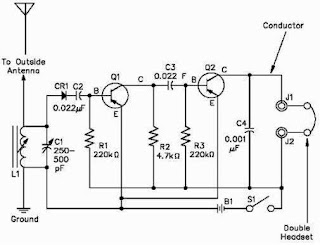Figure 1 Schematic Diagram

As an example, let us look at a schematic diagram of a two-transistor radio circuit (Figure 1). This diagram, from left to right, shows the components in the order they are used to convert radio waves into sound energy. By using this diagram it is possible to trace the operation of the circuit from beginning to end. Due to this important feature of schematic diagrams, they are widely used in construction, maintenance, and servicing of all types of electronic circuits.

One-Line Diagram

The one-line, or single-line, diagram shows the components of a circuit by means of single lines and the appropriate graphic symbols. One-line diagrams show two or more conductors that are connected between components in the actual circuit. The one-line diagram shows all pertinent information about the sequence of the circuit, but does not give as much detail as a schematic diagram. Normally, the one-line diagram is used to show highly complex systems without showing the actual physical connections between components and individual conductors. As an example, Figure 2 shows a typical one-line diagram of an electrical substation.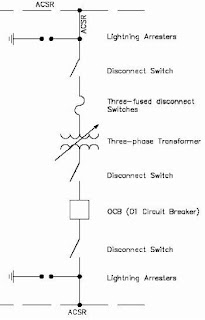Figure 2 One-Line Diagram

Block Diagram

A block diagram is used to show the relationship between component groups, or stages in a circuit. In block form, it shows the path through a circuit from input to output (Figure 3). The blocks are drawn in the form of squares or rectangles connected by single lines with arrowheads at the terminal end, showing the direction of the signal path from input to output. Normally, the necessary information to describe the stages of components is contained in the blocks.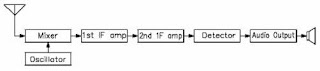Figure 3 Block Diagram

Wiring Diagram

A wiring diagram is a very simple way to show wiring connections in an easy-to-follow manner. These types of diagrams are normally found with home appliances and automobile electrical systems (Figure 4). Wiring diagrams show the component parts in pictorial form, and the components are identified by name. Most wiring diagrams also show the relative location of component parts and color coding of conductors or leads.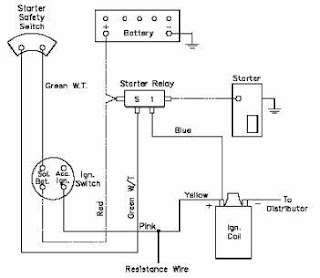Figure 4 Wiring Diagram

Resistivity

Resistivity is defined as the measure of the resistance a material imposes on current flow. The resistance of a given length of conductor depends upon the resistivity of that material, the length of the conductor, and the cross-sectional area of the conductor, according to Equation (1).

R = rhoL / A……….(1)

where

R = resistance of conductor, ohm
rho= specific resistance or resistivity cm-ohm/ft
L = length of conductor, ft
A = cross-sectional area of conductor, cm

The resistivity(rho) allows different materials to be compared for resistance, according to their nature, without regard to length or area. The higher the value of rho, the higher the resistance. Table 1 gives resistivity values for metals having the standard wire size of one foot in length and a cross-sectional area of 1 cm.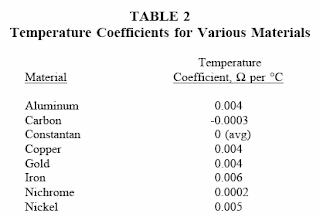Temperature Coefficient of Resistance

Temperature coefficient of resistance,(alpha), is defined as the amount of change of the resistance of a material for a given change in temperature. A positive value of alpha indicates that R increases with temperature; a negative value of alpha indicates R decreases; and zero alpha indicates that R is constant. Typical values are listed in Table 2.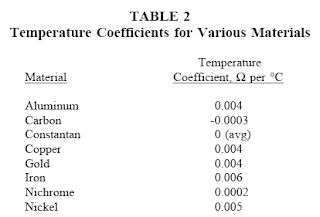For a given material, aplha may vary with temperature; therefore, charts are often used to describe how resistance of a material varies with temperature. An increase in resistance can be approximated from equation (2).

Rt = R0+ Ro(alpha.delta.T)………………(2)

where

Rt = higher resistance at higher temperatures
Ro = resistance at 20oC
alpha= temperature coefficient
dT = temperature rise above 20oC

Electric Circuit

Each electrical circuit has at least four basic parts: (1) a source of electromotive force, (2) conductors, (3) load or loads, and (4) some means of control. In Figure 5, the source of EMF is the battery; the conductors are wires which connect the various component parts; the resistor is the load; and a switch is used as the circuit control device.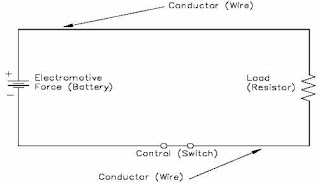Figure 5 Closed Circuit

A closed circuit (Figure 5) is an uninterrupted, or unbroken, path for current from the source (EMF), through the load, and back to the source.

An open circuit, or incomplete circuit, (Figure 6) exists if a break in the circuit occurs; this prevents a complete path for current flow.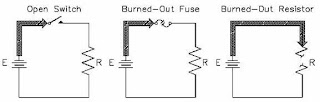Figure 6 Open Circuit

A short circuit is a circuit which offers very little resistance to current flow and can cause dangerously high current flow through a circuit (Figure 7). Short circuits are usually caused by an inadvertent connection between two points in a circuit which offers little or no resistance to current flow. Shorting resistor R in Figure 7 will probably cause the fuse to blow.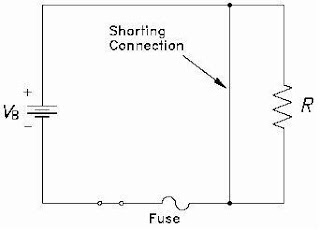Figure 7 Short Circuit

Series Circuit

A series circuit is a circuit where there is only one path for current flow. In a series circuit (Figure 8), the current will be the same throughout the circuit. This means that the current flow through R1 is the same as the current flow through R2 and R3.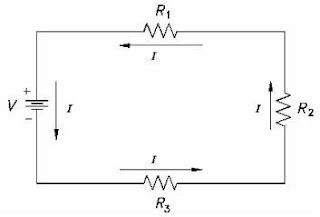Figure 8 Series Circuit

Parallel Circuit

Parallel circuits are those circuits which have two or more components connected across the same voltage source (Figure 9). Resistors R1, R2, and R3 are in parallel with each other and the source. Each parallel path is a branch with its own individual current. When the current leaves the source V, part I1 of IT will flow through R1; part I2 will flow through R2; and part I3 will flow through R3. Current through each branch can be different; however, voltage throughout the circuit will be equal.

V = V1 = V2 = V3.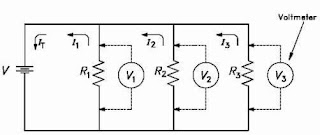Figure 9 Parallel Circuit

Equivalent Resistance

In a parallel circuit, the total resistance of the resistors in parallel is referred to as equivalent resistance. This can be described as the total circuit resistance as seen by the voltage source. In all cases, the equivalent resistance will be less than any of the individual parallel circuit resistors. Using Ohm’s Law, equivalent resistance (REQ) can be found by dividing the source voltage (V) by the total circuit current (IT), as shown in Figure 9.

REQ = V / It

Summary

The important information concerning basic DC circuits is summarized below. There are four types of circuit diagrams.
- Schematic diagram
- One-line diagram
- Block diagram
- Wiring diagram

• Resistivity is defined as the measure of the resistance a material imposes on current flow.
• Temperature coefficient of resistance, (alpha), is defined as the amount of change of the resistance of a material for a given change in temperature.
• A closed circuit is one that has a complete path for current flow.
• An open circuit is one that does not have a complete path for current flow.
• A short circuit is a circuit with a path that has little or no resistance to current flow.
• A series circuit is one where there is only one path for current flow.
• A parallel circuit is one which has two or more components connected across the same voltage source.
• Equivalent resistance is the total resistance of the resistors in parallel.

### DC Sources

When most people think of DC, they usually think of batteries. In addition to batteries, however, there are other devices that produce DC which are frequently used in modern technology.

Batteries

A battery consists of two or more chemical cells connected in series. The combination of materials within a battery is used for the purpose of converting chemical energy into electrical energy. To understand how a battery works, we must first discuss the chemical cell. The chemical cell is composed of two electrodes made of different types of metal or metallic compounds which are immersed in an electrolyte solution. The chemical actions which result are complicated, and they vary with the type of material used in cell construction. Some knowledge of the basic action of a simple cell will be helpful in understanding the operation of a chemical cell in general. In the cell, electrolyte ionizes to produce positive and negative ions (Figure 1, Part A). Simultaneously, chemical action causes the atoms within one of the electrodes to ionize.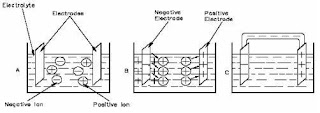Figure 1 Basic Chemical Battery

Due to this action, electrons are deposited on the electrode, and positive ions from the electrode pass into the electrolyte solution (Part B). This causes a negative charge on the electrode and leaves a positive charge in the area near the electrode (Part C). The positive ions, which were produced by ionization of the electrolyte, are repelled to the other electrode. At this electrode, these ions will combine with the electrons. Because this action causes removal of electrons from the electrode, it becomes positively charged.

DC Generator

A simple DC generator consists of an armature coil with a single turn of wire. The armature coil cuts across the magnetic field to produce a voltage output. As long as a complete path is present, current will flow through the circuit in the direction shown by the arrows in Figure 2. In this coil position, commutator segment 1 contacts with brush 1, while commutator segment 2 is in contact with brush 2. Rotating the armature one-half turn in the clockwise direction causes the contacts between the commutator segments to be reversed. Now segment 1 is contacted by brush 2, and segment 2 is in contact with brush 1.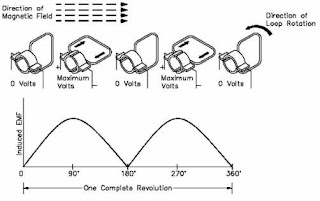Figure 2 Basic DC Generator

Due to this commutator action, that side of the armature coil which is in contact with either of the brushes is always cutting the magnetic field in the same direction. Brushes 1 and 2 have a constant polarity, and pulsating DC is delivered to the load circuit.

Thermocouples

A thermocouple is a device used to convert heat energy into a voltage output. The thermocouple consists of two different types of metal joined at a junction (Figure 3).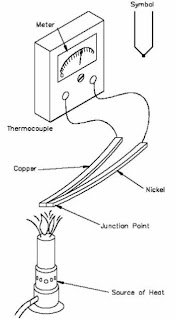Figure 3 Production of a DC Voltage Using a Thermocouple

As the junction is heated, the electrons in one of the metals gain enough energy to become free electrons. The free electrons will then migrate across the junction and into the other metal. This displacement of electrons produces a voltage across the terminals of the thermocouple. The combinations used in the makeup of a thermocouple include: iron and constantan; copper and constantan; antimony and bismuth; and chromel and alumel. Thermocouples are normally used to measure temperature. The voltage produced causes a current to flow through a meter, which is calibrated to indicate temperature.

Rectifiers

Most electrical power generating stations produce alternating current. The major reason for generating AC is that it can be transferred over long distances with fewer losses than DC; however, many of the devices which are used today operate only, or more efficiently, with DC. For example, transistors, electron tubes, and certain electronic control devices require DC for operation. If we are to operate these devices from ordinary AC outlet receptacles, they must be equipped with rectifier units to convert AC to DC. In order to accomplish this conversion, we use diodes in rectifier circuits. The purpose of a rectifier circuit is to convert AC power to DC. The most common type of solid state diode rectifier is made of silicon. The diode acts as a gate, which allows current to pass in one direction and blocks current in the other direction. The polarity of the applied voltage determines if the diode will conduct. The two polarities are known as forward bias and reverse bias.

Forward Bias

A diode is forward biased when the positive terminal of a voltage source is connected to its anode, and the negative terminal is connected to the cathode (Figure 4A). The power source’s positive side will tend to repel the holes in the p-type material toward the p-n junction by the negative side. A hole is a vacancy in the electron structure of a material. Holes behave as positive charges. As the holes and the electrons reach the p-n junction, some of them break through it (Figure 4B). Holes combine with electrons in the n-type material, and electrons combine with holes in the p-type material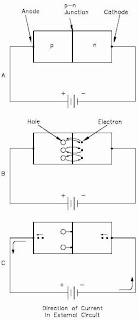Figure 4 Forward-Biased Diode

When a hole combines with an electron, or an electron combines with a hole near the p-n junction, an electron from an electron-pair bond in the p-type material breaks its bond and enters the positive side of the source. Simultaneously, an electron from the negative side of the source enters the n-type material (Figure 4C). This produces a flow of electrons in the circuit.

Reverse Bias

Reverse biasing occurs when the diode’s anode is connected to the negative side of the source, and the cathode is connected to the positive side of the source (Figure 5A). Holes within the p-type material are attracted toward the negative terminal, and the electrons in the n-type material are attracted to the positive terminal (Figure 5B). This prevents the combination of electrons and holes near the p-n junction, and therefore causes a high resistance to current flow. This resistance prevents current flow through the circuit.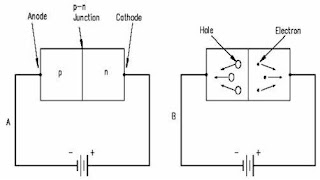Figure 5 Reverse-Biased Diode

Half-Wave Rectifier Circuit

When a diode is connected to a source of alternating voltage, it will be alternately forward-biased, and then reverse-biased, during each cycle of the AC sine-wave. When a single diode is used in a rectifier circuit, current will flow through the circuit only during one-half of the input voltage cycle (Figure 6). For this reason, this rectifier circuit is called a half-wave rectifier. The output of a half-wave rectifier circuit is pulsating DC.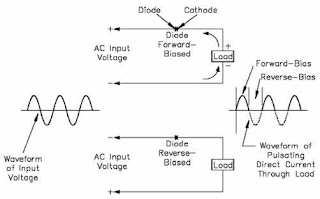Figure 6 Half-Wave Rectifier Full-Wave Rectifier Circuit

A full-wave rectifier circuit is a circuit that rectifies the entire cycle of the AC sine-wave. A basic full-wave rectifier uses two diodes. The action of these diodes during each half cycle is shown in Figure 7.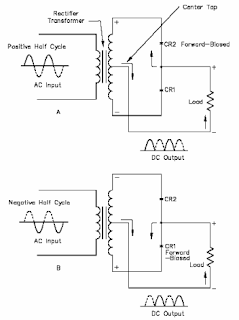Figure 7 Full-Wave Rectifier

Another type of full-wave rectifier circuit is the full-wave bridge rectifier. This circuit utilizes four diodes. These diodes’ actions during each half cycle of the applied AC input voltage are shown in Figure 8. The output of this circuit then becomes a pulsating DC, with all of the waves of the input AC being transferred. The output looks identical to that obtained from a full-wave rectifier (Figure 7).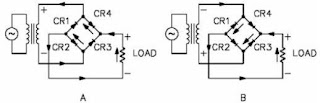Figure 8 Bridge Rectifier Circuit

Summary

The important information concerning DC sources is summarized below. There are four common ways that DC voltages are produced.
- Batteries
- DC Generators
- Thermocouples
- Rectifiers

• Thermocouples convert energy from temperature into a DC voltage. This voltage can be used to measure temperature.
• A rectifier converts AC to DC. There are two types of rectifiers.
- Half-Wave rectifiers
- Full-Wave rectifiers
• Half-wave rectifiers convert the AC to a pulsating DC and convert only onehalf of the sine wave.
• Full-wave rectifiers convert the AC to a pulsating DC and convert all of the sine wave.